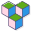CppBuzz# Java program to check if nubmer is a prime number

``````public class Main {

public static void main(String[] args) {
// Prime Number : the number which is divisible by 1 and itself.
// 2,3,5,7,11,13...........etc.
// 1 is not a prime number

int num = 3;
boolean flag = false;

for(int i=2; i<=num/2; i++) {
if(num % i == 0) {
flag = true;
break;
}
}
if(!flag) {
System.out.println(num + " is a prime number");
}
else {
System.out.println(num + " is not a prime number");
}
}

}``````